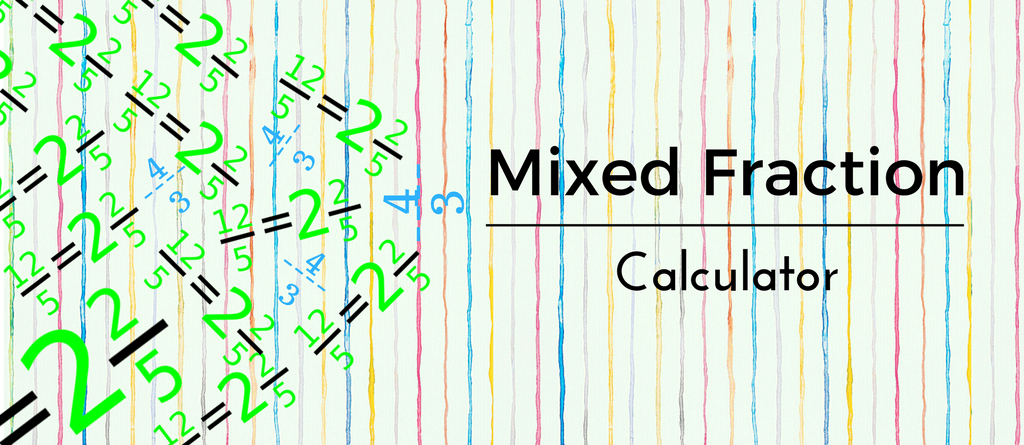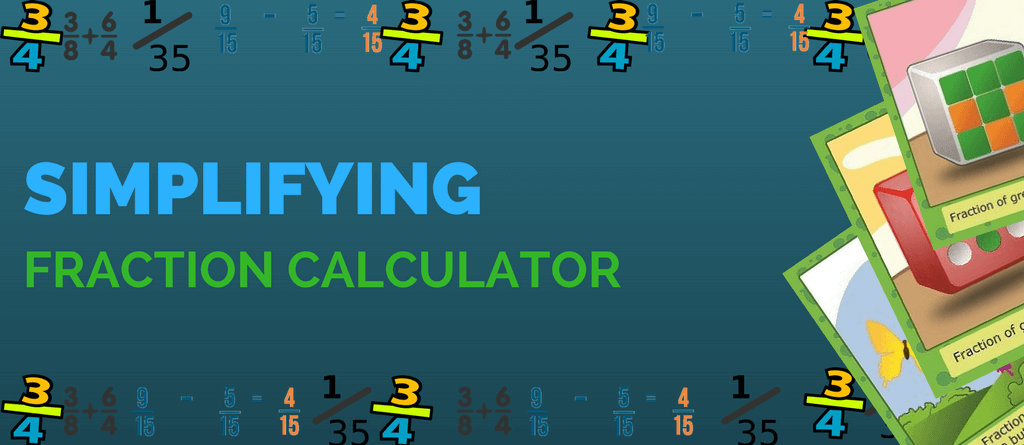Online Mixed Fraction Calculator With Steps

Mixed up about mixed fractions? Worry not. This online mixed fraction calculator is here to help. Just put the numbers in the spaces below for adding or subtracting mixed fractions. Yes! It is that simple. So, why wait? Let’s get started. Mixed Fraction Calculator Addition Let’s take an example: 2 1 + 3 1 4… Read more »Online Fraction Calculator With Steps – Add Subtract Multiply Divide

Online Fraction Calculator Simple! Put the numbers in the spaces below and choose the mathematical symbol from the drop-down menu to get the answer. See the step by step solution for your fraction sum by clicking on ‘hint’. Trouble understanding fractions? See the formula and step – by – step explanation of each operation in… Read more »Simplify Fractions Calculator With Steps

Got a big scary looking fraction to solve? No need to worry, this online fraction calculator will help you in reducing/simplifying your fraction problem in no time. NowÂ simplifying fractions is as easy as solving 2 + 2 on your head. ThisÂ simplify fractions calculator helps you to simplify fractions in a jiffy. What’s the wait for?… Read more »